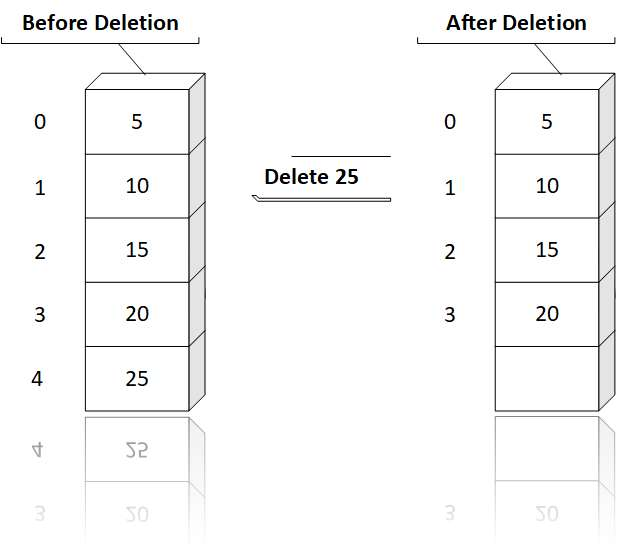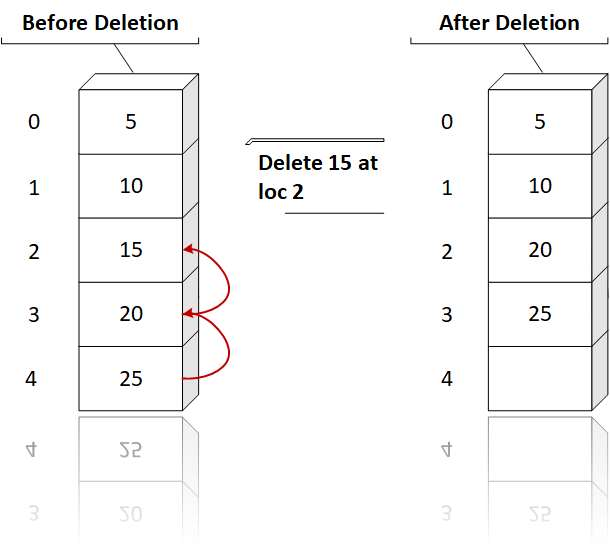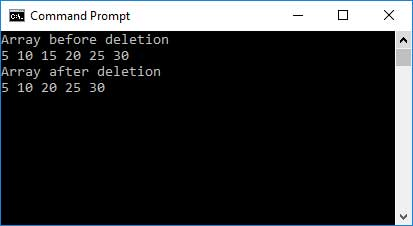# Array C Programing

Answer: Deletion refers to the operation of removing an element from existing list of elements. After deletion the size of the linear array is decreased by the factor of 1. Like insertion, deletion of an element from the end of array can be done very easily.

However, to delete an element from any other location, the elements are to be moved upward in order to fill up the location vacated by the removed element. Deletion can be done either at the end of array or at a given position.

Deletion can be done either at the end of array or at a given position.

Deletion at End:

Deletion at the end of array is very simple, we have to just find the last element and remove it.Deletion at a given position:

Deletion at a given position is a time consuming and expensive process as results in shifting the array elements upward in order to fill the vacant position.Code:

``````
// C program to implement delete operation in a
// unsorted array
#include

// To search a key to be deleted
int findElement(int arr[], int n,
int key);

// Function to delete an element
int deleteElement(int arr[], int n,
int key)
{
// Find position of element to be deleted
int pos = findElement(arr, n, key);

if (pos == - 1)
{
return n;
}

// Deleting element
int i;
for (i = pos; i < n - 1; i++)
arr[i] = arr[i + 1];

return n - 1;
}

// Function to implement search operation
int findElement(int arr[], int n, int key)
{
int i;
for (i = 0; i < n; i++)
if (arr[i] == key)
return i;

return - 1;
}

// Driver code
int main()
{
int i;
int arr[] = {5, 10, 15, 20, 25,30};

int n = sizeof(arr) / sizeof(arr);
int key = 15;

printf("Array before deletion\n");
for (i = 0; i < n; i++)
printf("%d ", arr[i]);

n = deleteElement(arr, n, key);

printf("\nArray after deletion\n");
for (i = 0; i < n; i++)
printf("%d ", arr[i]);

return 0;
}

``````

Output: